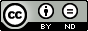### A New Generalized Entropy Measure and its Properties

Mohammad Javid Dar, Saima M. Sofi, Mirza A. K. Baig

#### Abstract

In this research article, a new two parametric measure of Entropy  and its corresponding code word length  has been developed. The developed measures are the generalizations to some well known existing measures. Besides, some noiseless coding theorems for discrete noiseless channel have been developed, and the results thus obtained have been verified with the support of an numerical example. Also, at the end of this research article, a comparative study in terms of  monotonic behavior  among the proposed entropy   , Matahi’s entropy  and Tsallis entropy  together  with their respective average code word length measures have been made and graphically displayed.

#### Keywords

Information, Shannon’s Entropy, Coding theory, Prefix Code, Average code-word Length, Kraft’s Inequality, Holder’s Inequality, Shannon Fanno Codes and Noiseless Coding theorem.

PDF

#### References

Baig M.A.K. and Javid M., : Some New Generalizations of Fuzzy Average Codeword Length and Their Bounds, American Journal of Applied Mathematics and Statistics, Volume 2, No. 2, pp. 73 – 76.

Campbell, L.L. : A coding theorem and Renyi’s entropy, Information and Control, Vol. 8, pp. 423-429.

Havrada, J. H. and Charvat, F. : Quantification methods of classificatory processes, the concepts of structural  entropy, Kybernetika, Vol.3, pp. 30-35..

Kapur, J. N. : A generalization of Campbell’s noiseless coding theorem, Jour. Bihar Math, Society, Vol.10, pp.1-10.

Kapur, J. N. : Entropy and Coding, Mathematical Science Trust Society, New Delhi.

Kraft, L.J. : A device for quantizing grouping and coding amplitude modulates pulses. M.S. Thesis, Department of of Electrical Engineering, MIT, Camridge.

Mathai, A.M. and Rathie, P.N. : Basic Concept in Information Theory and Statistics. Wiley Eastern Limited, New Delhi.

Renyi, A. : On measures of entropy and information. Proceedings 4th Berkeley Symposium on Mathematical Statistics and Probability, Vol.1, pp.541-561.

Shannon, C. E. : A mathematical theory of communication. Bell System Technical Journal, Vol.27, pp.379-423, 623-659.

Shannon, C. E. : Coding theorems for a discrete source with a fidelity criterion. In IRE National Convention Record, Part 4, pp142-163,

Sharma, B.D. and Taneja, I. J.: Entropies of type,  and other generalized measures of information theory, Mathematika, Vol.22, pp. 205-215.

Tsalli’s C. : Possible Generalization of Boltzmann-Gibbs statistics, Vol. 52, pp. 479-487.

DOI: https://doi.org/10.24203/ajfam.v7i3.6029

### Refbacks

• There are currently no refbacks.

Copyright (c) 2019 Asian Journal of Fuzzy and Applied Mathematics